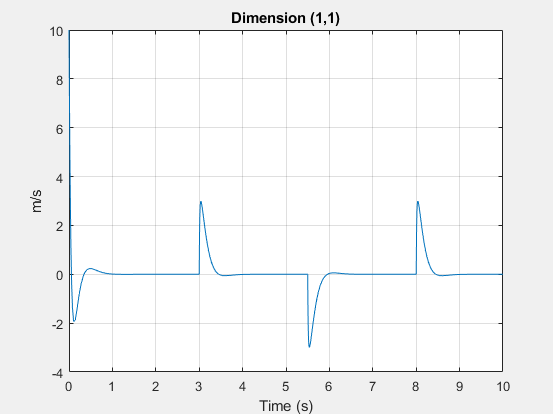# plot

Plot logged simulation series values against time

## Syntax

``fh = plot(series)``
``fh = plot(series,Name,Value)``

## Description

example

````fh = plot(series)` plots the simulation series values along the `y`-axis, with time along the `x`-axis. `fh` is a structure of handles to the resulting figures. `series` is a `simscape.logging.Series` object. `series` must include a full identifier path to the series, starting with the workspace log variable name.```

example

````fh = plot(series,Name,Value)` lets you customize the plot by using one or more `Name,Value` pair arguments. For example, specify '`time`' followed by a 1x2 vector, ```[start_time end_time]```, to plot only the data within this time range.```

## Examples

collapse all

Plot velocity of port R of a Translational Spring block.

Open the Mass-Spring-Damper with Controller example model:

`ssc_mass_spring_damper_control`

This example model has data logging enabled for the whole model, with the Workspace variable name parameter set to `simlog_ssc_mass_spring_damper_control`.

Simulate the model to log the simulation data:

`sim('ssc_mass_spring_damper_control');`

Plot velocity of port R of the Translational Spring block `Spring`.

`plot(simlog_ssc_mass_spring_damper_control.Spring.R.v.series);`Customize the previous plot using the name-value pair arguments.

This command plots the velocity of port R of the Translational Spring block in mm/s and only within the time range between 2 and 7 seconds.

```plot(simlog_ssc_mass_spring_damper_control.Spring.R.v.series,'units','mm/s','time',[2 7]); ```## Input Arguments

collapse all

Simulation data to plot, specified as a `simscape.logging.Series` object or a homogeneous cell array of such objects. `series` must include a full identifier path to the series, starting with the workspace log variable name.

The table describes the resulting plots based on the type of the `series` argument:

 Scalar `Series` object Plots the simulation series values along the `y`-axis, with time along the `x`-axis. Nonscalar `Series` object Plots each dimension of the series values on a different axis in the same figure window. Cell array of `Series` objects Plots all series objects with commensurate units on the same axis (superimposed), and each dimension for a nonscalar series on a different axis in the same figure window.The input arguments are binned based on commensurate units. For each bin, all `Series` objects with the same dimension as the first `Series` object in that bin are plotted and others are ignored.

### Name-Value Arguments

Specify optional pairs of arguments as `Name1=Value1,...,NameN=ValueN`, where `Name` is the argument name and `Value` is the corresponding value. Name-value arguments must appear after other arguments, but the order of the pairs does not matter.

Before R2021a, use commas to separate each name and value, and enclose `Name` in quotes.

Example: ```fh = plot(simlog.Translational_Spring.R.v.series,'units','mm/s')``` plots velocity of port R of the Translational Spring block in `mm/s`.

Plot legend, specified as the comma-separated pair consisting of '`names`' and a cell array of character vectors or string scalars. The number of elements in the cell array must be same as the number of elements of `series`.

By default, plots have no legend.

Time range for plotting the data, specified as the comma-separated pair consisting of '`time`' and a 1x2 vector, ```[start_time end_time]```, in seconds.

`[]` plots all data.

Units for plotting the data, specified as the comma-separated pair consisting of '`units`' and a unit name, or a cell array of unit names. Unit names must appear inside single quotes (`''`) or double quotes (`""`). Specified units must be commensurate with the units of the series values.

## Output Arguments

collapse all

Handles to the resulting plot figure windows, returned as a cell array.

## Version History

Introduced in R2010b